# AP Calculus AB Practice Test 17

### Test Information10 questions20 minutes

Calculator Disallowed

1. If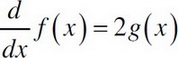and if h(x) = x3, then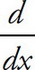f(h(x)) =

2.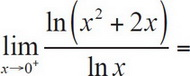3.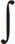(2x - 5)3dx =

4.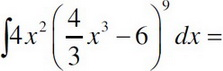5. Find the average value f(x) = 3x2sinx3 of on the interval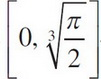.

6. Find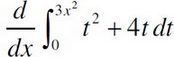.

7.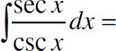8. What is the area between y = x3 and y = x ?

9. Find the volume of the region bounded by y = (x - 5)3, the x-axis, and the line x = 10 as it is revolved around the line x = 2. Set up, but do not evaluate the integral.

10.3x2(x3 - 3)7dx =